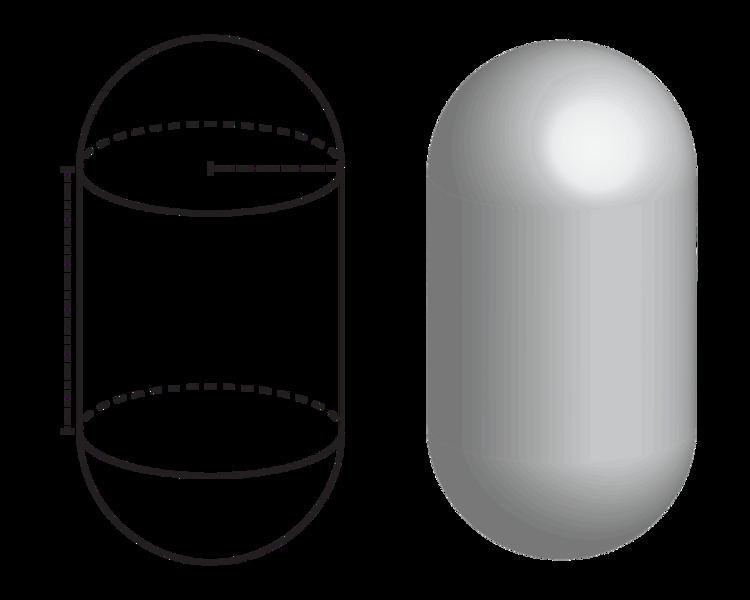# Capsule (geometry)

Updated on
Covid-19A capsule (from Latin capsula, "small box or chest"), or stadium of revolution, is a basic three-dimensional geometric shape consisting of a cylinder with hemispherical ends. Another name for this shape is spherocylinder.

The shape is used for containers for pressurised gasses and for pharmaceutical capsules.

## Formulas

The volume of a capsule is calculated by the formula V = π r 2 ( 4 3 r + a ) where r is the radius of the cylinder and hemispheres and a is the height of the cylindrical part.

The surface area formula is S A = 2 π r ( 2 r + a ) . .

## References

Similar Topics
La Damigella di Bard (film)
Jake Marketo
Mark Sampson
Topics

 B i Link H2
 L